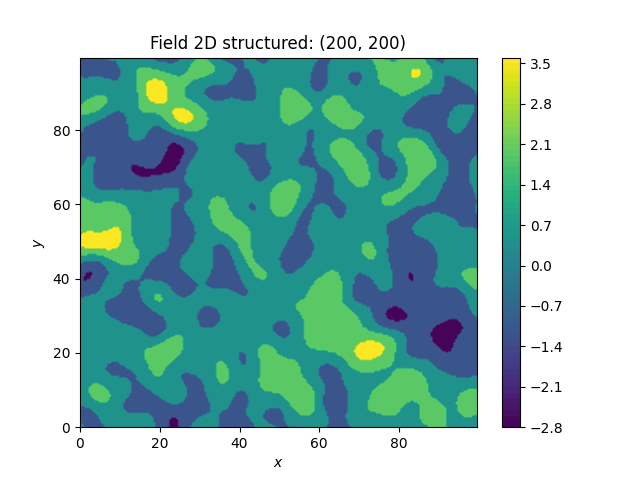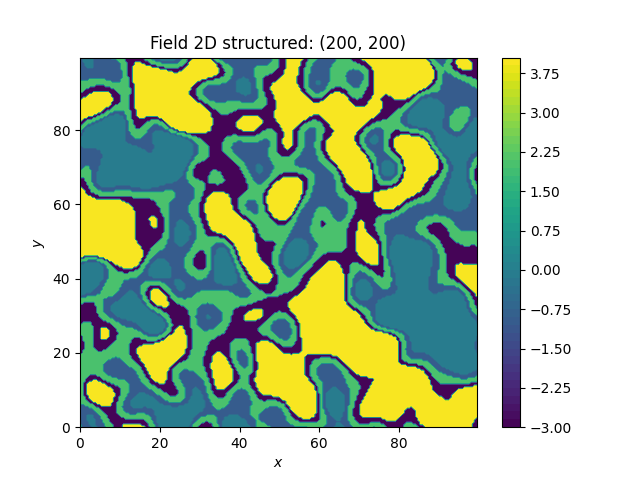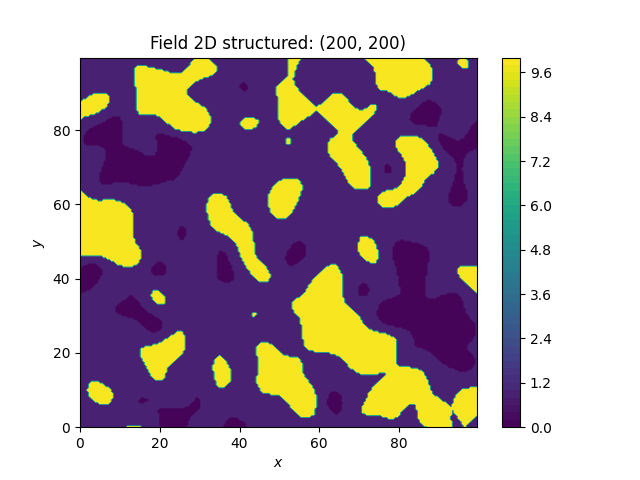# Discrete fields¶

Here we transform a field to a discrete field with values. If we do not give thresholds, the pairwise means of the given values are taken as thresholds. If thresholds are given, arbitrary values can be applied to the field.

•••```import numpy as np
import gstools as gs

# structured field with a size of 100x100 and a grid-size of 0.5x0.5
x = y = np.arange(200) * 0.5
model = gs.Gaussian(dim=2, var=1, len_scale=5)
srf = gs.SRF(model, seed=20170519)

# create 5 equidistanly spaced values, thresholds are the arithmetic means
srf.structured([x, y])
discrete_values = np.linspace(np.min(srf.field), np.max(srf.field), 5)
gs.transform.discrete(srf, discrete_values)
srf.plot()

# calculate thresholds for equal shares
# but apply different values to the separated classes
discrete_values2 = [0, -1, 2, -3, 4]
srf.structured([x, y])
gs.transform.discrete(srf, discrete_values2, thresholds="equal")
srf.plot()

# user defined thresholds
thresholds = [-1, 1]
# apply different values to the separated classes
discrete_values3 = [0, 1, 10]
srf.structured([x, y])
gs.transform.discrete(srf, discrete_values3, thresholds=thresholds)
srf.plot()
```

Total running time of the script: ( 0 minutes 9.622 seconds)

Gallery generated by Sphinx-Gallery